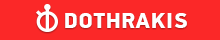GMAT Question of the Day - Daily to your Mailbox; hard ones only

 It is currently 26 Jun 2019, 11:22GMAT Club Daily Prep

Thank you for using the timer - this advanced tool can estimate your performance and suggest more practice questions. We have subscribed you to Daily Prep Questions via email.

Customized
for You

we will pick new questions that match your level based on your Timer History

Track

every week, we’ll send you an estimated GMAT score based on your performance

Practice
Pays

we will pick new questions that match your level based on your Timer History

Not interested in getting valuable practice questions and articles delivered to your email? No problem, unsubscribe here.If line segments AB and CD have lengths of 10 + 7 and 5 − 7 respective

Author Message
TAGS:

Hide Tags

Math ExpertV
Joined: 02 Sep 2009
Posts: 55803
If line segments AB and CD have lengths of 10 + 7 and 5 − 7 respective  [#permalink]

Show Tags00:00

Difficulty:15% (low)

Question Stats:84% (00:55) correct16% (00:53) wrongbased on 42 sessions

HideShow timer StatisticsIf line segments AB and CD have lengths of $$10 + \sqrt{7}$$ and $$5 − \sqrt{7}$$ respectively, AB is greater than CD by how much?

A. $$5 − 2\sqrt{7}$$

B. $$5 + 2\sqrt{7}$$

C. $$15 + 2\sqrt{7}$$

D. 5

E. 15

_________________
CEOP
Joined: 18 Aug 2017
Posts: 3947
Location: India
Concentration: Sustainability, Marketing
GPA: 4
WE: Marketing (Energy and Utilities)
If line segments AB and CD have lengths of 10 + 7 and 5 − 7 respective  [#permalink]

Show Tags

Bunuel wrote:
If line segments AB and CD have lengths of $$10 + \sqrt{7}$$ and $$5 − \sqrt{7}$$ respectively, AB is greater than CD by how much?

A. $$5 − 2\sqrt{7}$$

B. $$5 + 2\sqrt{7}$$

C. $$15 + 2\sqrt{7}$$

D. 5

E. 15

$$10 + \sqrt{7}$$ - ( $$5 − \sqrt{7}$$)

$$5 + 2\sqrt{7}$$

IMO B
_________________
If you liked my solution then please give Kudos. Kudos encourage active discussions.If line segments AB and CD have lengths of 10 + 7 and 5 − 7 respective   [#permalink] 25 Dec 2018, 08:12
Display posts from previous: Sort by

If line segments AB and CD have lengths of 10 + 7 and 5 − 7 respective